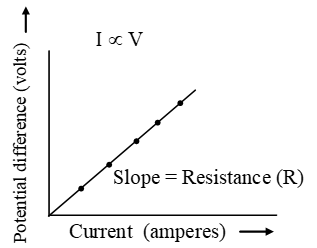# What is the Ohm’s law?

## What is the Ohm’s law?

1. The proportional relationship of the potential difference between the ends of an ideal conductor and the current passing through it is known as Ohm’s law.
2. Ohm’s law states that the current passing through an ideal conductor is directly proportional to the potential difference between its ends, provided that the temperature and other physical factors of the conductor are kept constant.
3. Definition: According to the Ohm’s law at constant temperature, the current flowing through a conductor is directly proportional to the potential difference across the conductor.
4. Thus, if I is the current flowing through a conductor and V is the potential difference (or voltage) across the conductor, then according to Ohm’s law.
I ∝ V (when T is constant)
$$I=\frac{V}{R}\text{ }…..\text{ (i)}$$
where R is a constant called the resistance of the conductor.
Equation (i) may be written as,
V = I × R                          …… (ii)
5. Unit of resistance:
The SI unit of resistance (R) is ohm. Ohm is denoted by the Greek letter omega (Ω).
$$\text{From Ohms law, }R=\frac{V}{I}$$
6. Now, if, V = 1 volt and I = 1 ampere
$$\text{Then, }R=\frac{\text{1}\,\text{volt}}{\text{1}\,\text{ampere}}$$
Thus, 1 ohm is defined as the resistance of a conductor which allows a current of 1 ampere to flow through it when a potential difference of 1 volt is maintained across it.
7. Results of Ohm’s law
Current flowing through a conductor is directly proportional to the potential difference across the conductor.8. When the potential difference in a circuit is kept constant, the current in inversely proportional to the resistance of the conductor.
$$I\propto \frac{1}{R}$$
9. The ratio of potential difference to the current is constant. The value of the constant is equal to the resistance of the conductor (or resistor).
$$\frac{V}{I}=R$$

## Ohm’s Law Problems with Solution

1. When a potential difference of 12 V is applied across a conductor, it is found that the current that passes through the conductor is 0.2 A. What is the resistance of the conductor?
Solution:
$$R = \frac{V}{I}$$
= 12/0.2
= 60 Ω
2. What is the potential difference across a light bulb of resistance 5 Ω when the current that passes through it is 0.5 A?
Solution:
V = I × R
= 0.5 × 5 = 2.5 V
3. A potential difference of 230 V is applied across an electric heater. A current of 5 A is flowing through the heating element of the heater.
(a) What is the resistance of the heating element?
(b) Can this electric heater be used in a country where the main supply is 110 V? Explain your answer.
Solution:
(a) $$R = \frac{V}{I}$$
= 230/5
= 46 Ω
(b) The heater can still be used but the heating effect will be very much lesser as the current passing through the heater is very much smaller. The current that passes through the heater is:
$$I = \frac{V}{R}$$
= 110/46
= 2.4 A
This value is smaller than 5 A.
4. An electric motor which has an internal resistance of 10 Ω can run at a certain speed when a current of 0.5 A passes through it. The speed of the motor increases when a potential difference twice as big as before is applied across it. What is the new current that passes through the motor?
Solution:
Potential difference across the motor when a current of 0.5 A is passing through:
V = IR = 0.5 x 10 = 5 V
When the potential difference is doubled
2 x 5 = I x 10
I = 1 A

## What is a superconductor made of?

1. Most materials have electrical resistance which leads to power loss and heating.
2. Many electrical applications require materials with very low resistance – the lower the better.
3. Power transmission lines, electromagnets and computer chips would be revolutionised by resistanceless materials.
4. In fact, under special conditions, materials with zero resistance can be made. For example, when mercury is cooled to a temperature of 4.15 K, its resistance suddenly dropped to zero. Mercury thus becomes a superconductor. This phenomenon is known as superconductivity.
5. Figure illustrates superconductivity. The resistance of a superconductor suddenly drops to zero as its temperature decreases below the critical temperature, Tc.6. A superconductor conducts electricity without loss of energy. Figure shows a permanent magnet floating above a superconductor. This is an effect of superconductivity that is due to a continuous flow of induced current.